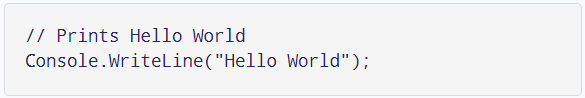# ITSE 1330 | C## Operators

## Foreword

There are many types of operators in C#. Some of the more common are: primary, unary, multiplicative, additive, relational, equality, and logical. In this chapter, we will review some of the more frequently used operators. The entire list can be viewed here.

## Operator Examples

Start a new C# project called Operators and code it as shown. Run the program to produce output in a command window. When reviewing the concepts, compare the source code with the output window. Note the comment on line 22. It is intentionaly the only comment in the code (except for the project name). I did this to make a point. Are comments in the other sections necessary? When the code is self-evident or self-explanatory the value of comments decreases. Does the Prints Hello World comment below add value? On the other hand, XML comments indicated by three slashes (///) can be used to produce documentation and may be used by some development teams. A good guideline might be, "If not sure if a comment will be beneficial, add a comment."Most of the operators in the code example are self-explanatory. For example, the addition and multiplication operators perform as you would expect. Some students may not be familiar with the modulus (or modulo) operator which is the percent % sign. When used in an expression, it returns the remainder. For instance, 17 % 5 will return a remainder of 2. Lines 25-36 demonstrate the familiar mathematical operators.

We have used the dot operator "." shown on lines 19, 23, 38, etc. since the early code examples. It is used to access members of an object or class. In our current code, the dot operator is being using to access the color property of the ConsoleColor class. The dot operator is known as a primary operator. Other primary operators such as the array operators [] and the function operators f() will be covered in subsequent chapters.

Lines 40-47 show the unary operators (++, --, !). They are called unary because only one operand is required. For instance, on line 41, myInt3 is the only operand. Whereas on line 33, there are two operands (17 and 3). The unary operators have two modes: prefix and postfix. The prefix mode (operator precedes) performs the operation on the variable first and then returns the value. The postfix mode (operator succeeds or follows) returns the original value and then performs the operation. For instance, on line 41, myInt3 will be incremented by 1 and then "returned" which is why 112 is returned.

Notice line 42 uses the postfix mode. That is, the ++ operator follows the operand (myInt3 in this case). The current value of myInt3 is 112 and this is returned (or supplied) and then the increment operation occurs. Notice that line 43 prints the new value of myInt3 which is 113. Check your understanding of the prefix/postfix concepts with the unary subtraction operator. On line 47 the negation operator ! is shown. It reverses or negates a boolean value.

The next two groups of operators are the relational and equality operators. Modify the values and rerun the code to ensure you understand how those operators perform.

The logical operators are used to test multiple boolean conditions. & is the symbol for logical AND. | is the logical OR. The expression on line 61 is evaluating: "is 1 > 2 AND 4 < 5?". The answer will be false since one is not greater than 2 even though four is less than 5. The OR evaluates to true if one OR the other operand is true. Code, review, and modify the logical examples to ensure understanding.

There are two more logical operators to mention that are called Conditional AND && and Conditional OR ||. These operators are like their simpler counterparts AND and OR but also provide a feature known as "short-circuit evaluation". For &&, this means that if the first operand (the left side) is false, the right side will not be considered since both must be true. Likewise for ||, if the left operand is true, there is no need to evaluate the right operand.

An indispensable operator that we use on most lines of code is the assignment operator = where we are assigning something, or the result of an expression, to a variable on the left. As you can see in the code example, it is the most common operator. The assignment operator also has shorthand variations known as compound assignment. (As an aside, compound assignment operators are implemented as lambda expressions in C# but that is not necessary to understand to use the operators and is an intermediate-level topic not covered in this course.) With compound assignment, multiple operations are being performed. Lines 82, 84, 86, and 88 demonstrate compound assignment operators. Compare the lines with the output shown.

Look at line 82 and the corresponding output. The += operator takes the operand on the left and adds it to the operand on the right. Can you guess what is happening? Line 82 can be read as "myInt = myInt + 222". So, myInt starts as 111, adds 222, to get 333 which is confirmed by the output. Addition and assignment are the two operations being performed on line 82. Subtraction, multiplication, and division examples are also provided.

## Operators Program.csOutput of the code is shown. Use it to confirm your understanding of the operators in C#. The operators covered are common to most modern programming languages. For example, if you have learned the operators in C++ or Java, those described here will be very familiar.

## Output Operators## What's Next?

Recall that the three primary constructs in all of programming are: sequence, selection, repetition. In the next chapter, we will begin making decisions/selections in our code.